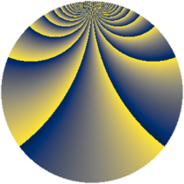# Properties

 Label 760.2.ccLevel $760$ Weight $2$ Character orbit 760.cc Rep. character $\chi_{760}(61,\cdot)$ Character field $\Q(\zeta_{18})$ Dimension $480$ Newform subspaces $1$ Sturm bound $240$ Trace bound $0$

# Related objects

## Defining parameters

 Level: $$N$$ $$=$$ $$760 = 2^{3} \cdot 5 \cdot 19$$ Weight: $$k$$ $$=$$ $$2$$ Character orbit: $$[\chi]$$ $$=$$ 760.cc (of order $$18$$ and degree $$6$$) Character conductor: $$\operatorname{cond}(\chi)$$ $$=$$ $$152$$ Character field: $$\Q(\zeta_{18})$$ Newform subspaces: $$1$$ Sturm bound: $$240$$ Trace bound: $$0$$

## Dimensions

The following table gives the dimensions of various subspaces of $$M_{2}(760, [\chi])$$.

Total New Old
Modular forms 744 480 264
Cusp forms 696 480 216
Eisenstein series 48 0 48

## Trace form

 $$480q + 6q^{4} + 6q^{6} + O(q^{10})$$ $$480q + 6q^{4} + 6q^{6} - 6q^{10} - 6q^{16} - 24q^{26} + 24q^{30} - 60q^{32} + 84q^{34} - 144q^{36} - 30q^{38} + 24q^{41} + 186q^{42} - 126q^{44} + 30q^{46} + 114q^{48} - 240q^{49} - 18q^{52} - 90q^{54} - 12q^{60} + 72q^{62} + 12q^{64} - 156q^{66} - 78q^{68} - 72q^{70} - 84q^{72} - 48q^{73} - 132q^{74} - 132q^{76} - 60q^{78} - 48q^{80} - 24q^{81} + 30q^{82} - 36q^{84} - 30q^{86} - 48q^{88} - 102q^{90} + 84q^{92} - 24q^{94} - 156q^{96} - 144q^{98} + O(q^{100})$$

## Decomposition of $$S_{2}^{\mathrm{new}}(760, [\chi])$$ into newform subspaces

Label Dim. $$A$$ Field CM Traces $q$-expansion
$$a_2$$ $$a_3$$ $$a_5$$ $$a_7$$
760.2.cc.a $$480$$ $$6.069$$ None $$0$$ $$0$$ $$0$$ $$0$$

## Decomposition of $$S_{2}^{\mathrm{old}}(760, [\chi])$$ into lower level spaces

$$S_{2}^{\mathrm{old}}(760, [\chi]) \cong$$ $$S_{2}^{\mathrm{new}}(152, [\chi])$$$$^{\oplus 2}$$